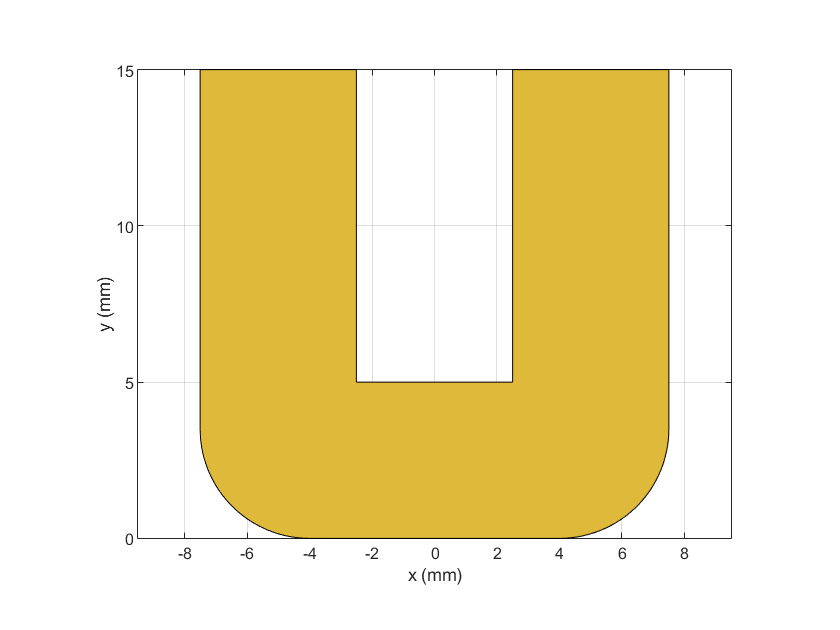# ubendCurved

Create U-bend with curved edges on X-Y plane

Since R2021b

## Description

Use the `ubendCurved` object to create a U-bend with curved edges on the X-Y plane.

## Creation

### Syntax

``bend = ubendCurved``
``bend = ubendCurved(Name=Value)``

### Description

example

````bend = ubendCurved` creates a U-bend with curved edges on the X-Y plane.```
````bend = ubendCurved(Name=Value)` sets Properties using one or more name-value arguments. For example, `ubendCurved(ReferencePoint=[1 1])` creates a U-bend with curved edges at the reference point `[1 1]`. Properties not specified retain their default values.```

## Properties

expand all

Name of the curved U-bend, specified as a character vector or a string scalar.

Example: `bend = ubendCurved(Name="ubendcurve1")`

Data Types: `char` | `string`

Reference point of the curved U-bend in Cartesian coordinates, specified as a two-element vector.

Example: `bend = ubendCurved(ReferencePoint=[1 2])`

Data Types: `double`

Length of the curved U-bend in meters, specified as a three-element vector of positive elements.

Example: `bend = ubendCurved(Length=[0.0050 0.0020 0.0050])`

Data Types: `double`

Width of the curved U-bend in meters, specified as a three-element vector of positive elements.

Example: `bend = ubendCurved(Width=[0.0040 0.0040 0.0040])`

Data Types: `double`

Radius of the corner in meters, specified as a positive scalar.

Example: `bend = ubendCurved(CurveRadius=0.0025)`

Data Types: `double`

## Object Functions

 `add` Boolean unite operation on two RF PCB shapes `and` Shape1 & Shape2 for RF PCB shapes `area` Calculate area of RF PCB shape in square meters `intersect` Boolean intersection operation on two RF PCB shapes `mesh` Change and view mesh properties of metal or dielectric in PCB component `minus` Shape1 - Shape2 for RF PCB shapes `plus` Shape1 + Shape2 for RF PCB shapes `rotate` Rotate RF PCB shape about defined axis `rotateX` Rotate RF PCB shape about x-axis `rotateY` Rotate RF PCB shape about y-axis and angle `rotateZ` Rotate RF PCB shape about z-axis `subtract` Boolean subtraction operation on two RF PCB shapes `scale` Change size of RF PCB shape by fixed amount `show` Display PCB component structure or PCB shape `translate` Move RF PCB shape to new location

## Examples

collapse all

Create a curved U-bend with default properties.

`curvedubend = ubendCurved`
```curvedubend = ubendCurved with properties: Name: 'myCurvedubend' ReferencePoint: [0 0] Length: [0.0150 0.0050 0.0150] Width: [0.0050 0.0050 0.0050] CurveRadius: 0.0035 ```

View the shape.

`show(curvedubend)`## Version History

Introduced in R2021b